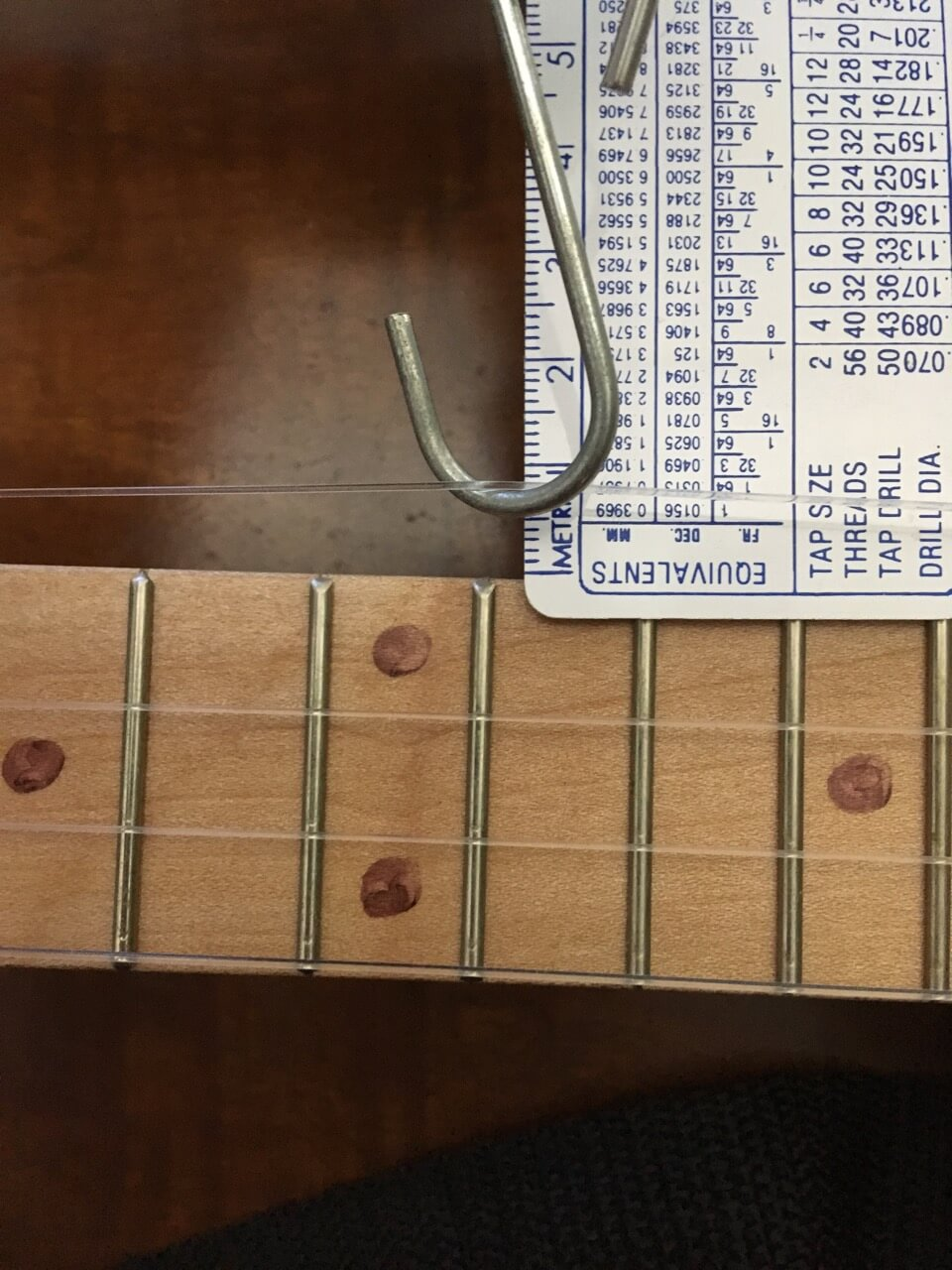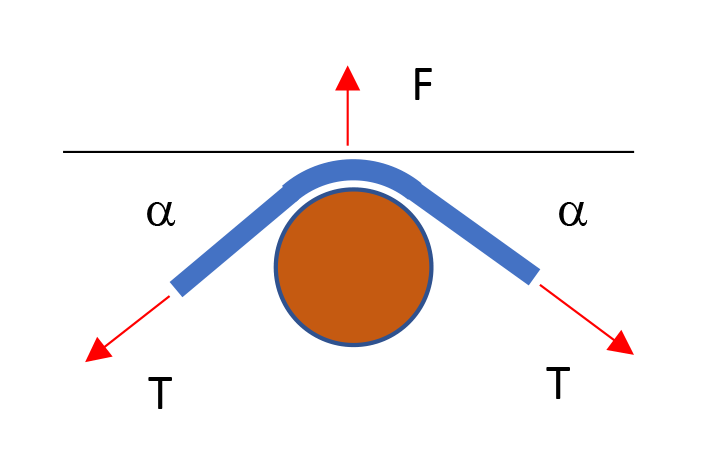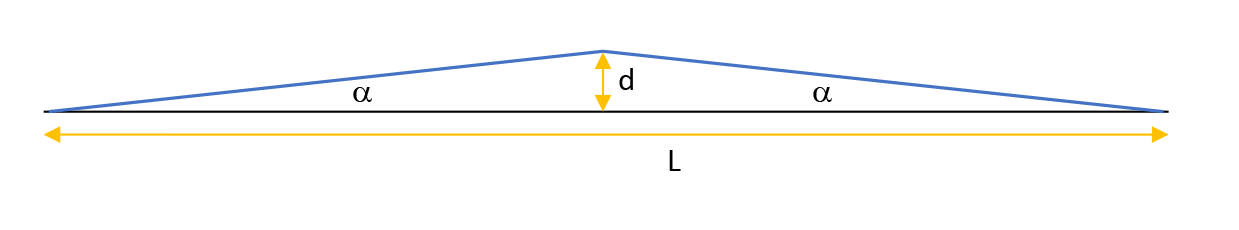# How to measure string tension easilyThey say that “high tension” strings pull harder on the neck of a guitar than “normal” or “light” tension. You have tested tension with your fingers and indeed some strings seem to have more tension than others, but how much, exactly? How can I tell whether the tension of one of my strings is too high or too low? I’ve run into this sort of discussion in several forums (like this one, or this other one, or still this one) and nobody seems to have the answer, yet you know that “your fingers tell you.” In this article, I translate that qualitative method into one that will give you a pretty accurate number, and explain the math behind it.

The basis of the method is exactly how you test tension with your fingers: you grab the string by the middle and pull it sideways. If it pulls a lot, the tension is “low,” but if it doesn’t budge, the tension is “high.” Very good, now let’s throw some simple instruments to get a number. Here’s what you need:

1.A ruler that is thin enough to slide under the strings at midpoint (12th fret).
2. A classroom-style dynamometer or spring scale—essentially, a calibrated spring—like that in the picture at right. Here’s a link on Amazon.And here is what you do to measure the tension of, say, a string in your guitar:

1. Slide the ruler under the string, next to the 12th fret (scale midpoint, if fretless), so the ruler is perpendicular to the string and the string is at the zero. Now hook the scale to the string at the midpoint (12th fret), and pull sideways a little so you get a decent reading on the scale. Record both the scale reading and the string displacement as measured on the ruler. On the left is a picture showing how I did it with one of my recent projects (fluorocarbon string on a cigar box guitar).
2. If the scale length is L, the displacement is d, and the spring scale reading is F, then the string tension is T = (F  L ) / (4 d)For instance, on my guitar I have a scale length of 21 inches = 525 mm. When pulling sideways to get a displacement of 10 mm, the spring scale read 3.3 Newtons. Therefore string tension is T = (3.3 N x 525 mm) / (4 x 10 mm) = 43.31 Newtons = 9.73 lb. This is a little loose because these strings are supposed to be tightened to a little over 10 lb.

This is the proof of the formula. The picture above shows a cross-section of the scale hook, pulling the blue string with force F. Since the string is itself pulling from both sides with tension T, but at a shallow angle alpha (same on both sides since the hook is at midpoint), balance of forces says: F = 2 T sin (alpha).The angle alpha is obtained from the geometry, shown in this other picture. You can see that tan(alpha) = d / (L/2). But since the angle is small, tan(alpha) is approximately equal to sin(alpha). Therefore sin(alpha) = 2d/L, and then T = F / (2sin(alpha)) = F / (2 x 2d/L) = FL/4d

I hope you find this little trick useful.

## 4 thoughts to “How to measure string tension easily”

1.Paul says:

I believe this measures the tension of the bent string. The more sensitive the scale the less you have to bend and the more accurate the un-bent tension would be. Maybe take a few measurements at different displacements. Probably is a formula that includes higher order terms that would give the exact undisplayed tension. Probably close enough anyway 🙂

2.Ronaldo says:

Fantastic post, Paco Ruiz! Thank you.

3.Chris Beatty says:

Great stuff. I was thinking this should be possible and found your post. I have two nerdy edits that don’t really add much. 1) The exact trigonometric solution is T= F/ sin (arctan(2*d/L)). I ran your numbers and got the same answer to three digits. So your approximations are fine. 2) I believe Paul above is correct that this gives the answer for the deflected string. If the deflection is small, it should be close. A more exact approach (in calculus speak) would be to say that T equals the limit of the above equation as d goes to zero. I tried using deflection of 1″, then 1/2″, then 1/4″. The values were decreasing a little. I would say that using a small deflection (~1/4″ or 6mm) and a delicate scale should give a pretty close result. Thanks for posting.

1.Paco Ruiz says:

You are right, strictly speaking one should use the exact trigonometric functions, which would remain valid even if the displacement is not so small. But there is another reason why the displacement has to be small, and this is that the tension will increase as the string is deflected. This effect, which allows a player to “bend” a note, will be particularly strong with metal strings.

This site uses Akismet to reduce spam. Learn how your comment data is processed.